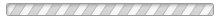Using a Planimeter (Area Measuring Tool) with Drawing Scales - EngineerSupply

# Using a Planimeter (Area Measuring Tool) with Drawing Scales

A planimeter will give correct results for "any" scale factor whatsoever, at least within the tolerance imposed by the operator and a small mechanical uncertainty.

In practice, an operator will trace an area on a plan with a known scale factor, and then multiply the "raw" planimeter reading by a constant, Ca, to get a corrected reading.

The constant Ca can be computed using an equation given in the instructions that come with each instrument. It is Ca = u*Sc^2 . "u" is the number of square inches per planimeter count, unique for each instrument and dependent on the arm length of that instrument. It is provided with each instrument shipped. Sc is the scale factor of the drawing to be measured.

For example, suppose an Operator is using a planimeter with its arm length set to medium length. He or she should refer to the calibration record that comes with the instrument and find that u is 0.017324 for a medium arm. The operator sees that the scale factor for his or her drawing is 1:400, meaning 1 unit = 400 units, where a unit can be an inch, a foot, or whatever. For this example the operator wants his answer to be in square feet so he must convert his 1:400 scale to the number of inches per foot. Since 1inch = 400 inches, it also equals 400 divided 12, or 33 1/3 feet. The hard part over, he or she uses the equation and finds that Ca equals 0.017324*(33 1/3)^2 , or 19.249.

If the operator has a digital readout, he simply keys in his scale factor of 19.249 and begins measuring. His answer will be in terms of square feet for each measurement. If the Operator has a mechanical planimeter, he or she must manually multiply each planimeter reading by
19.249 to get the number of square feet. If he or she stores the 19.249 in a calculator memory, this task becomes easier.

Some planimeters also give the value of u for metric measurements, that is, u is the number of square centimeters per planimeter count. In this case, the operator might have to convert the scale factor to give the number of centimeters per meter or whatever before computing Ca.

Disclaimer;  By reading this guide on our website, you agree to not hold anyone responsible for any misuse or misunderstandings that you may make. EngineerSupply.com does NOT assume any responsibility with providing this information.

Please Wait...